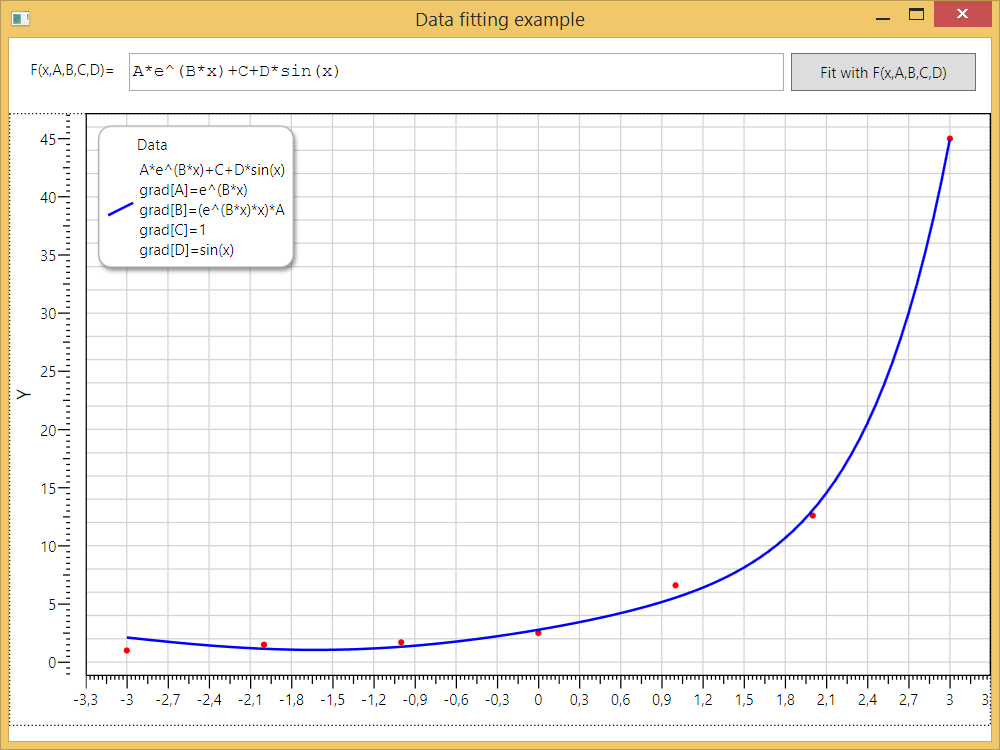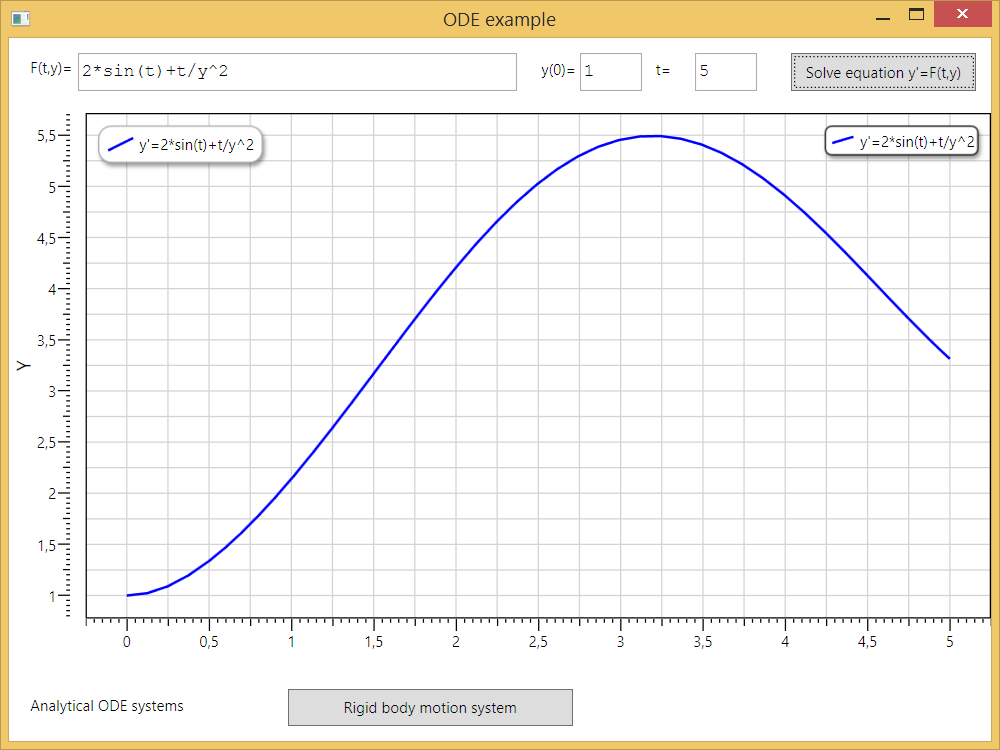NMath ANALYTICS – is a plugin for computational library NMath (.NET Math Library from CenterSpace, http://www.centerspace.net) that introduces analytical capabilities to it.

The advantages of using analytical capabilities are:

- Writing code without special classes for calculation delegates;

- Easily storing and transmitting data as strings;

- Convenient data presentation for developer and user;

- Automatically calculated derivatives for numerical algorithms.

- Parameterized problems solution.

NMath ANALYTICS provides integration between NMath and ANALYTICS libraries. The following extensions realized: Evaluation, Analysis, ODE and Optimization. Evaluation assembly realizes total analytical calculation engine for NMath double and complex vectors and matrices. Analysis assembly contains descendant classes for NMath functions those provide evaluation methods from analytical expressions, including Analytical Derivatives calculation. Optimization assembly contains Analytical descendants of parametrized functions for fitting data with arbitrary nonlinear functions set up in Analytical form. ODE assembly realizes special classes for solving ODE system set up in Analytical form.

NOTE: you need to download and install the NMath software (http://www.centerspace.net/nmath) for using NMath ANALYTICS library.

NMath ANALYTICS  Demo with Source code examples (VS2010 solution): NMathAnalytics.Demo.rar

(source code license include total NMath Analytics code

and all binaries of C# ANALYTICS 5.0 library)

SERVICES: you can order services directly from author: lrndlrnd@mail.ru

- Creating new library extensions for new math areas.

NMathAnalytics Evaluation

Evaluation assembly contains realization of special Translator class. This class registers analytical operations with NMath double and complex vectors and matrices.  Registered operations include:
- standard algebraic operators (+, -, *, /, ^);
- special vector operators (dot product);
- transcendental functions (sin, cos, exp, log and so on);
- basic special functions (abs, ceil and so on).

NMathAnalytics Analysis

Analysis assembly provides possibilities for differentiation and integration of functions set up in analytical form. These functions can be provided by user via simple string input. Realized Analytical function classes allows calculate Analytical derivative expressions that can be used then for evaluation purposes. For integration algorithms, the classes provide evaluation of analytical expression in any integration node.

NMathAnalytics Optimization

Optimization assembly introduces Analytical descendant classes of parametrized functions. Analytical classes allows construction of parametrized functions from string expressions (formulae) and then use evaluation methods for standard NMath numerical fitters and optimizers. More over, the classes provide Analytical form of gradient with respect to parameters. An example of data fitting with nonlinear 4-parametric function presented below on the picture:The approximation function, provided by user input, depends on four parameters A, B, C and D nonlinearly. Data fitting process done using Analytical gradient expression (shown on the graph description) automatically calculated by ANALYTICS derivative engine.

Advantages of using analytical functions for optimization purposes:
- No need to write special classes for calculation delegates;
- Easily storing data as strings (no need to make storage system for delegates);
- More convenient data presentation for developer and user;
- Automatically calculated derivatives (gradients) for numerical solvers.

NMathAnalytics ODE

Analytics Ordinary Differential Equation (ODE) extension allows using NMath ODE solvers for integration first order differential equation systems. The system set up as analytical expression set of the right part of differential equations.

An example for one equation solution presented on the picture below. The equation’s right part expression provided by user input:The example demonstrates advantages of using Analytical expressions for ODE:
- No need to write special classes for calculation delegates;
- Easily storing data as strings and transmit them via network;
- Allow users define their own differential equation systems;
- Write and solve parametrized systems.